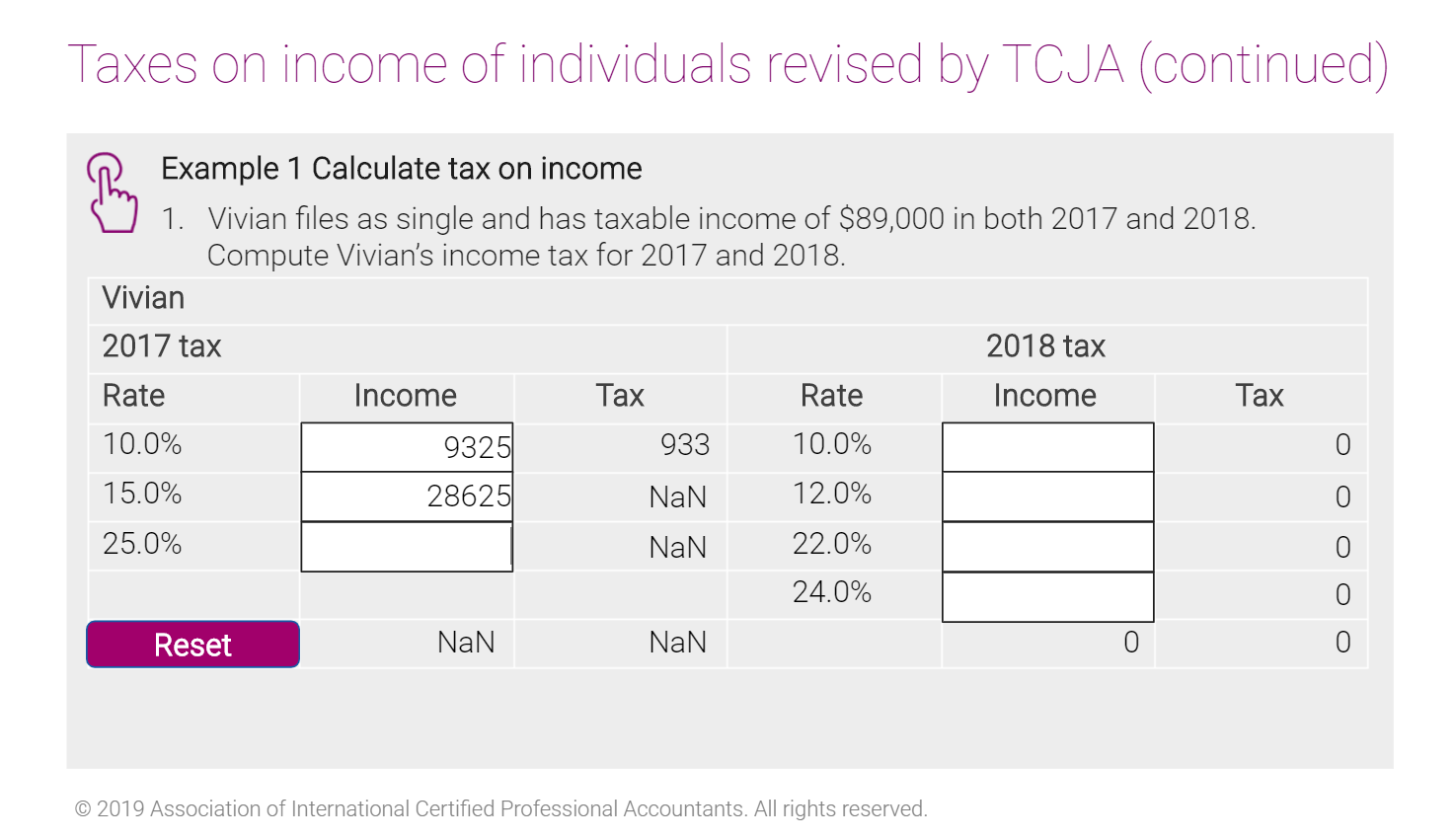# Rounding a variable (not results slide)

I have found articles regarding rounding (mainly multiply/divide by 1000000) but it doesnt work for me. I am not using a results slide. I am creating a conversion for Fahrenheit to Celsius and the math gives me a decimal. I have tried to incorporate the math above, but it still gives me the decimal. Does it only work on the results slide or is there another method?

###### 12 RepliesHello!  This is what I use to round - add a trigger to execute this Java.

I usually have a variable that contains the answer, then I pass it to another variable, then run it though this script.  NumberYearCalc is my answer that was passed.  And RNDofNumberYearCalc is my new variable that the rounded number will be passed to.

var player = GetPlayer();

var JSRounded=Math.round(player.GetVar("NumberYearCalc"));

player.SetVar("RNDofNumberYearCalc",JSRounded);I tried Mary's script on a project, and something weird's happening. Each number under Income is multiplied by the Rate to determine Tax. It works fine for the 1st row. It rounded the result up as desired. However, when I started inputting a number into the next field, NaN popped up. Everything worked fine before I used the javascript, so it's not a matter of mistakenly using a text field instead of a number field. Here's the screenshot, and I'm also attaching the story file.